# Illumination problem

(diff) ← Older revision | Latest revision (diff) | Newer revision → (diff)

The problem of determining the minimum number of directions of pencils of parallel rays, or number of sources, illuminating the whole boundary of a convex body. Letbe a convex body in an-dimensional linear space, let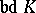and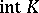be respectively its boundary and its interior, and assume that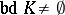. The best known illumination problems are the following.

1) Letbe a certain direction in. A pointis called illuminated from the outside by the directionif the straight line passing throughparallel topasses through a certain pointand if the direction of the vectorcoincides with. The minimum number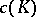of directions in the spaceis sought that is sufficient to illuminate the whole set.

2) Letbe a point of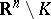. A pointis called illuminated from the outside by the pointif the straight line defined by the pointsandpasses through a point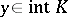and if the vectorsandhave the same direction. The minimum number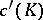of points fromis sought that is sufficient to illuminate the whole set.

3) Letbe a point of. A point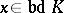is illuminated from within by the pointif the straight line defined by the pointsandpasses through a pointand if the vectors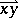and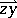have opposite directions. The minimum number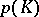of points fromis sought that is sufficient to illuminate the whole setfrom within.

4) A system of points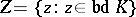is said to be fixing forif it possesses the properties: a)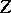is sufficient to illuminate the whole setfrom within; and b)does not have any proper subset sufficient to illuminate the setfrom within. The maximum number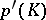of points of a fixing system is sought for the body.

Problem 1) was proposed in connection with the Hadwiger hypothesis (see ): The minimum number of bodieshomothetic to a boundedwith homothety coefficient,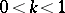, sufficient for covering, satisfies the inequality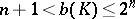, whereby the value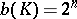characterizes a parallelepiped. For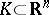bounded,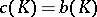. Ifis unbounded, then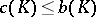, and there exist bodies such that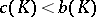or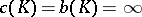(see ).

Problem 2) was proposed in connection with problem 1). Forbounded, the equality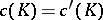holds. Ifis not bounded, then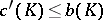and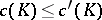. The numberfor any unboundedtakes one of the values 1, 2, 3, 4,(see ).

The solution of problem 3) takes the form: The numberis defined if and only ifis not a cone. In this case,whereby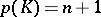characterizes an-dimensional simplex of the space(see ).

For problem 4) (see ), it has been conjectured that ifis bounded, the inequalityholds.

Every illumination problem is closely linked to a special covering of the body(cf. Covering (of a set)) (see ).

How to Cite This Entry:
Illumination problem. Encyclopedia of Mathematics. URL: http://encyclopediaofmath.org/index.php?title=Illumination_problem&oldid=47312
This article was adapted from an original article by P.S. Soltan (originator), which appeared in Encyclopedia of Mathematics - ISBN 1402006098. See original article